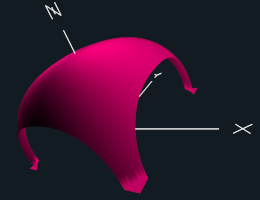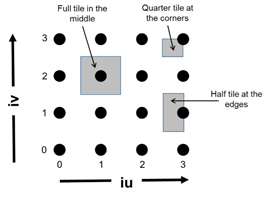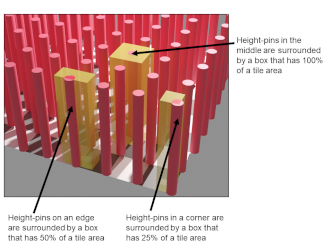## Project #2

### Due: April 26

This page was last updated: March 24, 2022Superquadric Surface with N = 2.5 and R = 1.2

### Introduction

We all know that the equation of a sphere centered at the origin is x2 + y2 + z2 = R2 But, there is something called a superquadric whose equation is xN + yN + zN = RN In this exercise, we will use parallel reduction to compute the volume of a superquadric using R=1.2. and N=2.5.

Using some number of subdivisions in both X and Y, NUMNODES x NUMNODES, take NUMNODES2 height samples.

We will think of each height sample as a pin extending vertically from the middle of a 2D tile. That really makes that height sample act as a volume where the tile is extruded vertically from the bottom to the top.

Think about a pin rising at each dot from the floor to the top of the superquadric surface. This pin's contribution to the overall volume is its height times the area of the tile underneath it.
Note: tiles along the edges will have only 1/2 the area of tiles in the middle.
Note: tiles in the corners will have only 1/4 the area of tiles in the middle. The volume contribution of each extruded height tile needs to be weighted accordingly. The logic of this is for you to figure out.

### Requirements

• Compute the total volume by using the given Height function. (Note that this will give you Z=0. to Z=Height. As the superquadric has a negative half in addition to a positive half, double the 0.-Height volume as your final answer.

• Use a variety of number of subdivisions (NUMNODES). Pick at least 8 different ones.

• Use a variety of number of threads (NUMT). You must use at least 1, 2, and 4.

• Record the data in units of something that gets larger as speed increases. Joe Parallel used "MegaHeights Computed Per Second", but you can use anything that makes sense.

• From the speed-up that you are seeing, use the "Inverse Amdahl's Law" to determine the Parallel Fraction for this application.

• From the Parallel Fraction, determine what maximum speed-up you could ever get, regardless of how many cores/threads you throw at this, even with a million cores.

• Your commentary write-up (turned in as a PDF file) should include:
1. Tell what machine you ran this on
2. What do you think the actual volume is?
3. Show the performances you achieved in tables and two graphs showing:
1. Performance as a function of NUMNODES with colored lines showing different NUMT values
2. Performance as a function of NUMT with colored lines showing different NUMNODES values
(See the example in the Project Notes.)
4. What patterns are you seeing in the speeds?
5. Why do you think it is behaving this way?
6. What is the Parallel Fraction for this application, using the Inverse Amdahl equation?
7. Given that Parallel Fraction, what is the maximum speed-up you could ever get?

### The Program Flow

The code below is printed in the handout to make it easy to look at and discuss. You can get this code in the file proj02.cpp.

In this code sample, NUMNODES is the number of nodes, or dots, subdividing the floor area. So, for example, NUMNODES=4 means that there are 4 dots on each side edge.Each node has some amount of tile space surrounding it:You could use a single for-loop over all the nodes that looks like this:

```
#pragma omp parallel for default(none) . . .
for( int i = 0; i < NUMNODES*NUMNODES; i++ )
{
int iu = i % NUMNODES;
int iv = i / NUMNODES;
float z = Height( iu, iv );
. . .
}

. . .
```

Or, you could also use the collapse OpenMP clause, where the (2) signifies how many nested for-loops to collapse into one for-loop:

```
#pragma omp parallel for collapse(2) default(none) . . .
for( int iv = 0; iv < NUMNODES; iv++ )
{
for( int iu = 0; iu < NUMNODES; iu++ )
{
float z = Height( iu, iv );
. . .
}
}
```

Note! The above pragma lines are not complete. You need to add more to them!

The code to evaluate the height at a given iu and iv is:

```
const float N = 2.5f;
const float R = 1.2f;

. . .

float
Height( int iu, int iv )	// iu,iv = 0 .. NUMNODES-1
{
float x = -1.  +  2.*(float)iu /(float)(NUMNODES-1);	// -1. to +1.
float y = -1.  +  2.*(float)iv /(float)(NUMNODES-1);	// -1. to +1.

float xn = pow( fabs(x), (double)N );
float yn = pow( fabs(y), (double)N );
float rn = pow( fabs(R), (double)N );
float r = rn - xn - yn;
if( r <= 0. )
return 0.;
float height = pow( r, 1./(double)N );
return height;
}
```

### The main Program

Your main program would then look something like this:

```
#define XMIN     -1.
#define XMAX      1.
#define YMIN     -1.
#define YMAX      1.

float Height( int, int );	// function prototype

int main( int argc, char *argv[ ] )
{
. . .

// the area of a single full-sized tile:
// (not all tiles are full-sized, though)

float fullTileArea = (  ( ( XMAX - XMIN )/(float)(NUMNODES-1) )  *
( ( YMAX - YMIN )/(float)(NUMNODES-1) )  );

// sum up the weighted heights into the variable "volume"
// using an OpenMP for-loop and a reduction:

?????
}
```